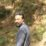Connect with us

# What is the Area of Parallelogram?Published

onIn mathematics, the area can be considered as the space or region a two-dimensional figure occupies. The area of a parallelogram can be defined as the space which is occupied by the given parallelogram. The formula given for it is, b * h where b is the base and h is the height of parallelogram respectively. The resultant value is always in square units. For example, if 4 cm is multiplied by 2 cm, the answer will be equal to 8 cm square units. In this article, we will try to cover some interesting aspects of a parallelogram and its area.

## Parallelogram and Its Types

There are various geometrical shapes in mathematics such as square, rectangle, rhombus, trapezium, and many others. Similarly, the parallelogram is a type of geometrical shape which is a type of quadrilateral. A parallelogram has four sides where the parallel and opposite sides are equal to each other. For example, if a parallelogram is ‘ABCD’, here AB is parallel to CD, and on opposite sides, AC is equal to BD. There are various classifications of the parallelogram namely, square, rhombus, and rectangle. All of these shapes possess distinctive properties. In the next paragraph, we may deal with the properties of parallelograms and their types.

## Properties of Parallelogram

As mentioned above, the parallelogram is a type of quadrilateral having parallel and opposite sides equal. In the next section, we may cover the various properties of parallelograms in a nutshell. Some of them are mentioned below:

1.  The opposite sides of a parallelogram are always equal and parallel.
2.  The angles opposite to each other are also equal in a parallelogram. The diagonal bisects the parallelogram into two triangles that are congruent to each other.
3. A parallelogram is categorized into three types. Such as Rhombus, Square, and Rectangle. Each of these shapes possesses its own properties on the basis of their nature.
4.  One common property about these shapes is that the diagonal of each shape bisects themselves respectively.

## Types of Parallelograms: Square, Rhombus, and Rectangle

The following is the types of parallelograms:

1. A square is a type of parallelogram which is bounded by four equal and parallel sides wherever ever angle measures about 90 degrees.
2. A type of parallelogram is a two-dimensional figure consisting of four sides and corners.
3.  This shape is considered as the special kind of parallelogram which has two pairs of parallel sides. It is known as Rhombus.

## Some Calculations Based on Area of Parallelogram

The mathematical formula given for the area of a parallelogram is ‘ b * h ‘ where b is denoted as base and h is defined as the height of the parallelogram. Let us solve some examples related to it so that you grasp the concept clearly.

Example 1: Calculate the area of a parallelogram, if the height and base is 6 cm and 7 cm respectively?

According to the question,

Base of the parallelogram = 6 cm

Height of the parallelogram = 7 cm

Using the formula of area of parallelogram = b * h

6 cm * 7 cm = 42 cm square units.

Therefore, the area of the parallelogram for the given height and base is equivalent to 42 cm square units.

Example 2: Calculate the area of a parallelogram, if the height and base are 9 cm and 6 cm respectively?

According to the question,

Base of the parallelogram = 9 cm

Height of the parallelogram = 6 cm

Using the formula of area of parallelogram = b * h

9 cm * 6 cm = 54 cm square units.

Therefore, the area of the parallelogram for the given height and base is equivalent to 54 cm square units.

If you want to learn about parallelogram and its area in a detailed, fun, and interactive manner, visit Cuemath and book a free session.